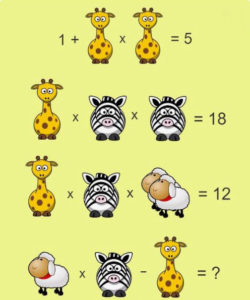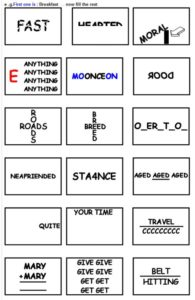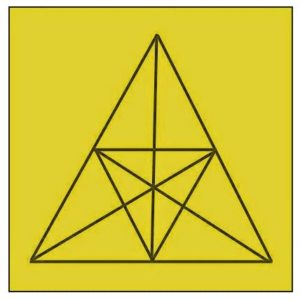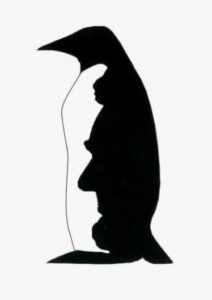Select Page

July 2018

## Did you try each several times?

Here are the answers to Brain Practice, July 2018

#### 3 Dogs – two giraffes = 0 BUT3 dogs + two giraffes = 12

Dog = 2
Giraffe = 3

So 2 + 3 = 5.

? = 5

NB: The value of the Giraffe changes for this second puzzle.

#### Did you try the more Difficult Challenge!1+ 2 Giraffes (G)= 5
5-1 = 4
4 divided by 2 =G
Giraffe =2

therefore,

2+ Z x Z = 18
2 + Z (squared) = 18
18 divided by 2 =9
Z (squared ) = 9
Square root of 9 = 3
Zebra=3

Giraffe x Zebra x 2 Sheep = 12
therefore
2 x 3 x 2S = 12
12 divided by 2 divided 3 =2
2S =2
2 divided by 2 = 1
Sheep = 1

Sheep x Zebra – Giraffe  = 1 +x 3 – 2 = 1

#### How Many Words of three or more letters (at least one five-letter word)

are, art, arts, aster, ate, ear, ears, east, eat, eats, era, eras, erst, rat, rate, rates, re-sat, rest, ret, rets, sate, sat, sea, sear, seat, sera, set, star, stare, tar, tare, tares, tars, taser, tea, tear, tears, teas, tsar

Any others??

Left to Right:Breakfast, Half-Hearted, Moral Support

Ready for Anything, Once in a Blue Moon, Back door

A Friend in Need, For Instance, Middle-Aged

Quite Right, Your Time is Up, Travel Overseas

Summary, Forgive and Forget, Hitting Below the Belt

#### Did you Solve these??

Forwards it is heavy, backwards it is not.
What is it?

THE WORD ‘TON’
——————-

How can you share 5 apples with 5 people and still have one left in the basket?

——————-
How do you make the number 66 one-and-a-half times bigger without doing any maths?

Turn the numerals upside down!

#### How Many Triangles?47

Explanation:
At first look, it seems pretty easy but on the contrary, it is a pretty tricky question. (Look at the three-dimensional scope of the image.)

Divide the triangle into three equal triangles (formed if the outer side is connected to the centre of the circle) and then count the number of triangles in each part by taking two or more parts together.

There are 4 non-overlapping and 3 overlapping triangles. 4 + 3 = 7 and 7 x 3 = 21

Next, if we take number of triangles by taking two parts together, there are 8 in total.
8 x 3 = 24

Lastly, there are 2 more triangles if all three parts are taken together. 47!

#### Penguin or President Optical IllusionDepending on how you look at it, you could see a flightless bird indigenous to cold climates or the 16th resident of the United States. Which do you see first in this president or penguin optical illusion…?

Many people see the profile of Abraham Lincoln first. Only after taking in the entire image does the penguin come in to focus. If you are able to switch back and forth between the two subjects in this president or penguin illusion quickly and easily, your cognitive flexibility is doing well!